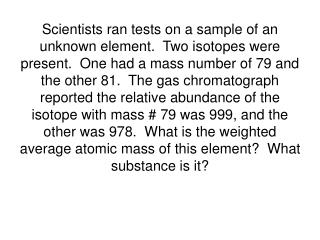DownloadDownload Presentation79 x 999 = 78921 81 x 978 = + 79218 158139 158139  1977 = 79.99

# 79 x 999 = 78921 81 x 978 = + 79218 158139 158139  1977 = 79.99

Download Presentation## 79 x 999 = 78921 81 x 978 = + 79218 158139 158139  1977 = 79.99

- - - - - - - - - - - - - - - - - - - - - - - - - - - E N D - - - - - - - - - - - - - - - - - - - - - - - - - - -
##### Presentation Transcript

1. Scientists ran tests on a sample of an unknown element. Two isotopes were present. One had a mass number of 79 and the other 81. The gas chromatograph reported the relative abundance of the isotope with mass # 79 was 999, and the other was 978. What is the weighted average atomic mass of this element? What substance is it?

2. 79 x 999 = 78921 81 x 978 = +79218 158139 158139  1977 = 79.99 The sample is bromine.

3. A professor’s class is weighted as follows: Tests = 80 % Quizzes = 5 % Lab Reports = 15% If a student has earned: 364/400 on tests 30/50 on quizzes 113/150 on lab reports • What is the student’s overall average for the course? • If the student earns an 88/100 on the next test, show how that affects the grade and explain why the grade moves the way it does, even though the test grade was higher than the overall average.

4. Question (a): 364/400 = 91% (Test - 80% of grade) 30/50 = 60% (Quiz - 5% of grade) 113/150 = 75.3% (Lab Report - 15% of grade) .8(91) + .05(60) + .15(75.3) = 87.1 % avg OR 80(91) + 5(60) + 15(75.3) = 87.1 % avg 100

5. Question (b): 452/500= 90.4% (Test - 80% of grade) 30/50 = 60% (Quiz - 5% of grade) 113/150 = 75.3% (Lab Report - 15% of grade) .8(90.4) + .05(60) + .15(75.3) = 86.6 % avg OR 80(90.4) + 5(60) + 15(75.3) = 86.6 % avg 100 The test grade was an 88%, which was higher than the overall average of 87.1%, so most students think their grade should go up. But because the test average was a 91%, this test brought that average down, and therefore brought down the overall grade instead of increasing the overall grade.